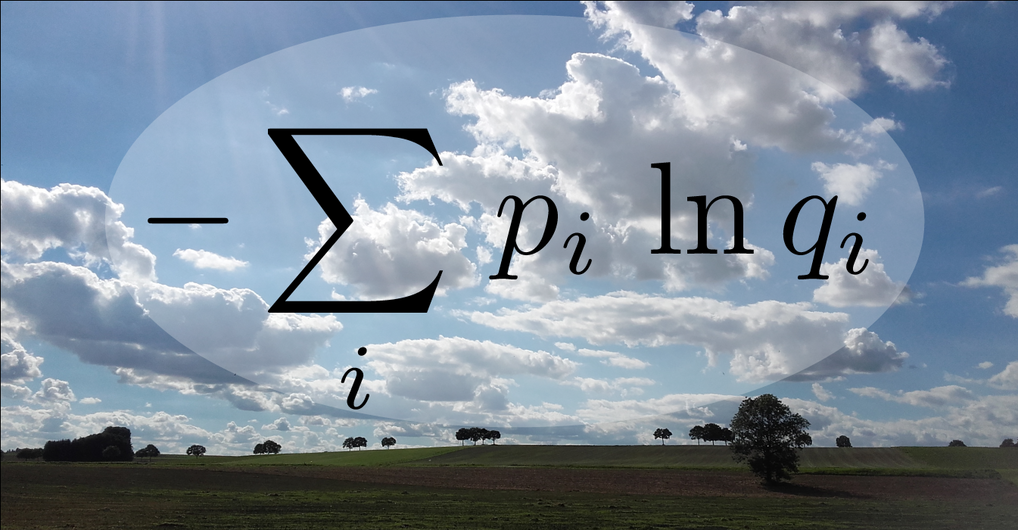# The embarrassment of false predictions - How to best communicate probabilities?

December 01, 2016

Complex predictions such as election forecasts or the weather reports often have to be simplified before communication. But how should one best simplify these predictions without facing embarrassment? In astronomical data analysis, researchers are also confronted with the problem of simplifying probabilities. Two researchers at the Max Planck Institute for Astrophysics now show that there is only one mathematically correct way to measure how embarrassing a simplified prediction can be. According to this, the recipient of a prediction should be deprived of the smallest possible amount of information.

Everybody knows this situation: The weather forecast predicts sunny weather, apparently with one hundred percent probability. You left your umbrella at home, and now you are left standing in the rain. This is not only annoying, but also embarrassing for the weather service. Did the weather people really not know better, or did they just communicate their knowledge imprecisely?

For efficient communication, statements have to be simplified. The weather service, for example, provides rounded probabilities for broad weather categories, even if the values used internally are much more complex. But how much simplification is tolerable before you risk embarrassment? And how do you measure the potential embarrassment of a prediction?

Reimar Leike and Torsten Enßlin at the Max Planck Institute for Astrophysics encountered this question while developing astronomical imaging methods. They show that there are only two requirements necessary to decide how good a simplification of a prediction is. First: if it is possible to communicate the precise probabilities, please do so. The second requirement is a bit more complex: The quality of a prediction depends only on the probability it assigned to the event that ultimately occurred. The simplified probabilities assigned to events which didn’t occur are irrelevant.The formula for the expected embarrassment of a prediction. The various possible events are labelled by i. For the weather forecast this could represent the possibilities "rain", "sunshine", etc. The original probabilities of these events are pi. The probabilities communicated in the prediction are qi. In case of simplification, these should be chosen in a way that the expected embarrassment is minimised. Ideally, the communicated probabilities are identical to the original probabilities, but often they are simplified or rounded. The concept of the embarrassment expected is closely related to the information concept of information theory, and clarifies how this should be used in the case of probability approximation. © T. Enßlin/MPA

To determine how to translate a complex probability into a simplified prediction, the researchers introduce a measure, which they call the embarrassment of the prediction. From the requirements above, they deduce mathematically that the embarrassment of a prediction is measured by the negative logarithm of the communicated probability of the event that ultimately occurred. This measure is often called the degree of surprise. A prediction can be called embarrassing if you tend to be surprised when trusting it.

As the event which will ultimately occur is still unknown at the time of the prediction, the expected surprise has to be estimated. All possible events have to be considered, taking into account both the embarrassment of the communicated prediction in case this event occurs, and its probability. The expected embarrassment of the simplified prediction should then be minimised by adapting the communication accordingly.

It thereby turns out that the communication should lack as little information as possible. This may sound obvious, however, it is a precise mathematical instruction for the simplification of probabilities. For example, this instruction implies that small probabilities are better rounded up than off. If the probability for rain is four percent, it is better to communicate this with ten percent rather than zero percent, even if the latter corresponds to the normal rounding rules. This is because the prediction of zero percent rain probability would be infinitely embarrassing in case of rain. According to the scale of the researchers, the occurrence of an impossible event (expressed by zero probability) corresponds to an infinitely great surprise (the negative logarithm of zero is infinite).

The work of Reimar Leike and Torsten Enßlin not only clarifies an academic question - in fact there is confusion about the optimal simplification of probabilities in the corresponding literature - but also has very useful consequences in many diverse areas, such as communication, astronomical imaging, and artificial intelligence. All these fields work with probabilities and occasionally have to simplify them - but without embarrassment, please.

## Other Interesting Articles

Go to Editor View